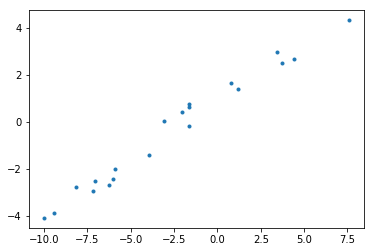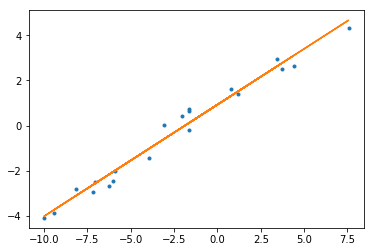# Linear algebra's approach to least squares¶

In :
%matplotlib inline
import matplotlib.pyplot as plt
import numpy as np
from scipy.linalg import solve, eig


When it comes to data $\{(x_i,y_i)\}$, the least squares problem is to find $f(x)=ax+b$ that best fits that data. That is we'd like to minimize $$\sum (ax_i + b - y_i)^2.$$ from the perspective of linear algebra, we'd like to minimize the squared norm $$\left\|\left(\begin{matrix} x_1 & 1 \\ x_2 & 1 \\ \vdots & \vdots \\ x_n & 1 \end{matrix}\right) \left(\begin{matrix} a \\ b \end{matrix} \right) - \left(\begin{matrix} y_1 \\ y_2 \\ \vdots \\ y_n \end{matrix}\right)\right\|^2.$$ More generally, we'd like to solve $A\vec{x} = \vec{b}$ in this least squares sense. As we learn in class, this can be done by solving the system $A^T A \vec{x}=A^T\vec{b}$.

Here's an example:

In :
np.random.seed(1)
xs = np.random.uniform(-10,10,20)
ys = [x/2 + 1 + np.random.normal(0,1/2) for x in xs]
plt.plot(xs,ys, '.')

Out:
[<matplotlib.lines.Line2D at 0x621bf2278>]In :
A = np.array([xs,np.ones(len(xs))]).transpose()
a,b = solve(A.transpose().dot(A), A.transpose().dot(ys))
a,b

Out:
(0.4939751982072998, 0.9304316886189519)
In :
def f(x): return a*x+b
fy = [f(x) for x in xs]
plt.plot(xs,ys, '.')
plt.plot(xs, fy)

Out:
[<matplotlib.lines.Line2D at 0x621c66a90>]Suppose we'd like to minimize $$p(x,y,z) = 10 x^2-10 x y-22 x z+2 x+6 y^2+4 y z-20 y+66 z^2-4 z+5.$$ Note that we can rewrite this as $$\left(\begin{matrix}x & y & z \end{matrix}\right) \left(\begin{matrix}10 & -5 & -11 \\ -5 & 6 & 2 \\ -11 & 2 & 66\end{matrix}\right) \left(\begin{matrix}x \\ y \\ z \end{matrix}\right) -2 \left(\begin{matrix}x & y & z \end{matrix}\right) \left(\begin{matrix}-1 \\ 10 \\ 2 \end{matrix}\right) +5.$$ If that matrix is positive definite, then the minminizer should be the solution of the following system:

In :
K = np.array([
[10,-5,-11],
[-5,6,2],
[-11,2,66]
])
solve(K,[-1,10,2])

Out:
array([1.58730159, 2.92063492, 0.20634921])

Unfortunately, it can be hard to tell if a symmetric matrix is positive definite. One way to tell is to try to row reduce it. If you can do so without row interchanges and using all positive pivots, then the matrix is positive definite. That's not too hard to see in this case. Another characterization is that all the eigenvalues are positive:

In :
eig(K)

Out:
array([68.21870698+0.j, 11.54070021+0.j,  2.24059281+0.j])

Of course, the matrix still needs to be symmetric.